### N Disposal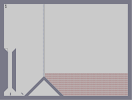Hover over the thumbnail for a full-size version.

Author Genius_of_the_System author:genius_of_the_system dda unrated 2005-11-23 3 more votes required for a rating. \$N Disposal#Genius_of_the_System#none#00000000000211111111150000000000000000000000000000000000031111111114000000000000000000000000000000000000000000000000000000000000000000000300000000000000000000035000000000000000000003500000000000000000000350000000000000000000035000000000000000000000240000000000000000000000240000000000000000000000240000000000000000000000240000000000000000000000200000000000000000000000000000000000000000000000000000000000000000000000000000000000000000000000000000000000000000000000000000000000000000000000000000000000000000000000000000000000000000000000000000000000000000000000000000000000000000000000000000000000000000000000000000000000000000000000000000000000000000000000000000000000000000000000000000000000000000000000000000000|5^36,36!2^60,564,1,0!2^132,564,0.707106781186547,-0.707106781186547!1^36,300!7^252,444,0!7^252,420,0!7^252,396,0!7^252,372,0!7^252,348,0!7^252,324,0!7^252,300,0!7^252,276,0!7^252,252,0!7^252,228,0!7^252,204,0!7^252,180,0!7^252,156,0!7^252,132,0!7^252,108,0!7^252,84,0!7^252,60,0!7^252,36,0!12^276,468!12^288,480!12^300,492!12^312,504!12^324,516!12^336,528!12^348,540!12^360,552!12^372,564!12^384,576!12^384,564!12^384,552!12^372,552!12^384,540!12^372,540!12^360,540!12^384,528!12^372,528!12^360,528!12^360,528!12^348,528!12^384,516!12^372,516!12^360,516!12^348,516!12^336,516!12^384,492!12^384,504!12^372,504!12^360,504!12^348,504!12^336,504!12^324,504!12^372,492!12^360,492!12^348,492!12^336,492!12^324,492!12^312,492!12^276,456!12^288,456!12^288,468!12^300,456!12^300,480!12^300,468!12^312,480!12^312,468!12^312,456!12^324,480!12^324,468!12^324,456!12^336,480!12^336,468!12^336,456!12^348,480!12^348,468!12^348,456!12^360,480!12^360,468!12^360,456!12^372,480!12^384,480!12^372,468!12^372,456!12^384,468!12^384,456!12^276,444!12^288,444!12^300,444!12^312,444!12^324,444!12^336,444!12^348,444!12^360,444!12^372,444!12^384,444!12^396,444!12^396,456!12^396,468!12^396,480!12^396,480!12^396,492!12^396,504!12^396,516!12^396,528!12^396,552!12^396,540!12^396,564!12^396,576!12^768,576!12^756,576!12^744,576!12^732,576!12^708,576!12^720,576!12^696,576!12^684,576!12^672,576!12^660,576!12^648,576!12^636,576!12^624,576!12^612,576!12^600,576!12^588,576!12^576,576!12^564,576!12^552,576!12^540,576!12^528,576!12^516,576!12^504,576!12^492,576!12^480,576!12^456,576!12^468,576!12^444,576!12^432,576!12^420,576!12^408,576!12^408,444!12^408,456!12^408,468!12^408,480!12^408,492!12^408,516!12^408,504!12^408,528!12^408,540!12^408,552!12^408,564!12^768,564!12^756,564!12^732,564!12^744,564!12^720,564!12^708,564!12^696,564!12^684,564!12^672,564!12^660,564!12^648,564!12^636,564!12^624,564!12^612,564!12^600,564!12^588,564!12^576,564!12^564,564!12^552,564!12^540,564!12^528,564!12^528,564!12^516,564!12^504,564!12^492,564!12^480,564!12^468,564!12^456,564!12^444,564!12^432,564!12^420,564!12^420,444!12^420,456!12^420,468!12^420,480!12^420,492!12^420,504!12^420,516!12^420,528!12^420,540!12^420,552!12^768,552!12^756,552!12^744,552!12^732,552!12^720,552!12^708,552!12^696,552!12^684,552!12^672,552!12^660,552!12^648,552!12^636,552!12^624,552!12^612,552!12^600,552!12^588,552!12^576,552!12^564,552!12^552,552!12^540,552!12^528,552!12^516,552!12^504,552!12^492,552!12^480,552!12^468,552!12^456,552!12^444,552!12^432,552!12^432,444!12^432,456!12^432,468!12^432,480!12^432,492!12^432,504!12^432,516!12^432,540!12^432,528!12^768,540!12^756,540!12^744,540!12^732,540!12^708,540!12^720,540!12^696,540!12^684,540!12^672,540!12^660,540!12^636,540!12^648,540!12^624,540!12^612,540!12^600,540!12^588,540!12^576,540!12^564,540!12^552,540!12^540,540!12^528,540!12^516,540!12^492,540!12^480,540!12^456,540!12^444,540!12^468,540!12^504,540!12^444,528!12^444,516!12^444,504!12^444,492!12^444,480!12^444,468!12^444,456!12^444,444!12^768,528!12^756,528!12^744,528!12^732,528!12^720,528!12^708,528!12^696,528!12^672,528!12^684,528!12^660,528!12^636,528!12^648,528!12^624,528!12^612,528!12^600,528!12^588,528!12^576,528!12^552,528!12^576,528!12^564,528!12^540,528!12^528,528!12^516,528!12^504,528!12^492,528!12^480,528!12^468,528!12^456,528!12^456,444!12^456,456!12^456,468!12^456,480!12^456,492!12^456,504!12^456,516!12^768,516!12^756,516!12^744,516!12^732,516!12^720,516!12^696,516!12^708,516!12^684,516!12^672,516!12^660,516!12^648,516!12^636,516!12^624,516!12^612,516!12^612,516!12^600,516!12^588,516!12^576,516!12^564,516!12^552,516!12^540,516!12^528,516!12^516,516!12^504,516!12^492,516!12^480,516!12^468,516!12^468,444!12^468,456!12^468,468!12^468,480!12^468,492!12^468,504!12^768,504!12^756,504!12^744,504!12^732,504!12^720,504!12^720,504!12^708,504!12^708,504!12^696,504!12^696,504!12^684,504!12^672,504!12^660,504!12^648,504!12^636,504!12^624,504!12^612,504!12^600,504!12^588,504!12^576,504!12^564,504!12^552,504!12^540,504!12^528,504!12^516,504!12^504,504!12^492,504!12^480,504!12^480,444!12^480,456!12^480,468!12^480,480!12^480,492!12^768,492!12^756,492!12^744,492!12^732,492!12^720,492!12^708,492!12^696,492!12^684,492!12^660,492!12^672,492!12^648,492!12^636,492!12^624,492!12^612,492!12^600,492!12^588,492!12^576,492!12^564,492!12^552,492!12^540,492!12^528,492!12^516,492!12^504,492!12^492,492!12^492,444!12^492,456!12^492,468!12^492,480!12^768,480!12^756,480!12^744,480!12^732,480!12^720,480!12^708,480!12^696,480!12^684,480!12^672,480!12^648,480!12^660,480!12^636,480!12^624,480!12^612,480!12^588,480!12^600,480!12^576,480!12^564,480!12^552,480!12^540,480!12^528,480!12^516,480!12^504,480!12^504,444!12^504,456!12^504,468!12^768,468!12^756,468!12^756,468!12^744,468!12^732,468!12^708,468!12^720,468!12^696,468!12^684,468!12^672,468!12^660,468!12^648,468!12^636,468!12^624,468!12^612,468!12^588,468!12^600,468!12^576,468!12^564,468!12^552,468!12^540,468!12^528,468!12^516,468!12^516,444!12^516,456!12^756,456!12^768,456!12^744,456!12^732,456!12^720,456!12^708,456!12^696,456!12^684,456!12^672,456!12^660,456!12^648,456!12^636,456!12^624,456!12^612,456!12^588,456!12^600,456!12^588,456!12^576,456!12^564,456!12^552,456!12^540,456!12^528,456!12^528,444!12^768,444!12^756,444!12^744,444!12^732,444!12^720,444!12^708,444!12^696,444!12^684,444!12^672,444!12^660,444!12^648,444!12^636,444!12^624,444!12^612,444!12^588,444!12^600,444!12^576,444!12^564,444!12^552,444!12^540,444# Yay! Smash N! I'm not crazy I'm just big boned.

## Other maps by this author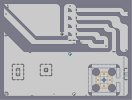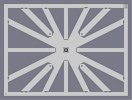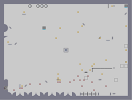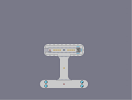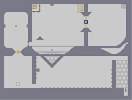Wacky World Sun Shine Out of the frying pan... Assention Jumping Castle